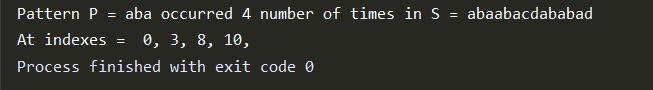## Rabin-Karp Algorithm

This is a pattern searching algorithm. Let’s say we have a string “S” and we have to find the occurrences of pattern “P” in “S”.

For Example →

S = ‘ababcde’

P = ‘ab’ // pattern we need to search for.

Ans - ‘ab’ occurs at 0th index of S

at 2nd index of S

Total number of occurrences = 2

(Note - We are assuming leln(S)>=len(P) else answer is 0).

Lets first see the naive algorithm for finding the number of occurrences and index of occurrences.

### Naive Approach -

We can iterate over every ith index of string S and check for the next len(p) characters if it matches with p or not. If it matches then incrementing the count and add the index i in the answer array.

Code In Python -

Output -Time Complexity -- O(len(S)*len(P))

## Rabin-Karp Approach -

This algorithm is based on finding the hash number for the pattern and then check if there exists a substring in the string S having the same hash number.

So let’s see what a simple hashing looks like -

Taking the values as -

a → 1

b → 2
.
.
.

z → 26

We can define a simple hash function as sum of values of characters in a string P.

For Example -

for P = “bcd” our hash function will give value = 2+3+4 = 9

But it is possible to have other string having the same hash number.

Take P = “ccc” value = 3+3+3 = 9

So we need to define our hash function so that we can minimize the number of collisions (i.e Two string having the same hash number).

Hash Function Given by Rabin Karp Algorithm -

(i) Let pr be any prime.

(ii) Mod be a large number to store hash number as modulo mod to avoid overflow.

For any string P of length k, we can have a hash number asWe can take mod and pr according to the constraints of the problem. Taking large mod will reduce the number of collisions. Moreover, for pr it is preferred to take a prime number larger than or equal to the length of the string to minimize the number of collisions.

Finding New Window Hash Value In O(1) →

We can find new window hash value by basic mathematics -

In moving to new window we just need to remove the first element and add the new element which will reduce val(S[first element of the window]) and add val(P[new element])*(pow(pr,len(P)) and then if we see it carefully we will see there is still an extra (pr) multiplied to all element so diving the current hash value now with pr will do out work.

Steps will be as follows(suppose we have curr hash value in currhashvalue) -

1. Currhashvalue -= val(P[first element of window])

2. Currhasshvalue += val(P[new element])*(pow(pr,len(P)))

3. Currhashvalue /= pr

4. Currhashvalue %=mod

Let’s implement the algorithm using some values -

Code In Python -

Output -Time Complexity -

O(len(S) + len(P) + numberofmatches * (len(P))

Average time complexity = O(len(S) + len(P))

By choosing optimal pr and mod values such that

the number of collisions gets reduced significantly gives

us the linear time complexity.

Worst case time complexity - O(len(P)*len(S))

The idea of the Rabin-Karp algorithm is widely used in plagiarism detection.

It is highly recommended to solve below problems, they are handpicked by Programmers Army:

Practice Problems :-